# Identify the odd one out using direction, position and number

In this worksheet, students will identify an image that does not fit in with the rest of the group. The focus will be on direction, position and number, so students will need to look at where shapes are, which direction they are facing, and how many there are.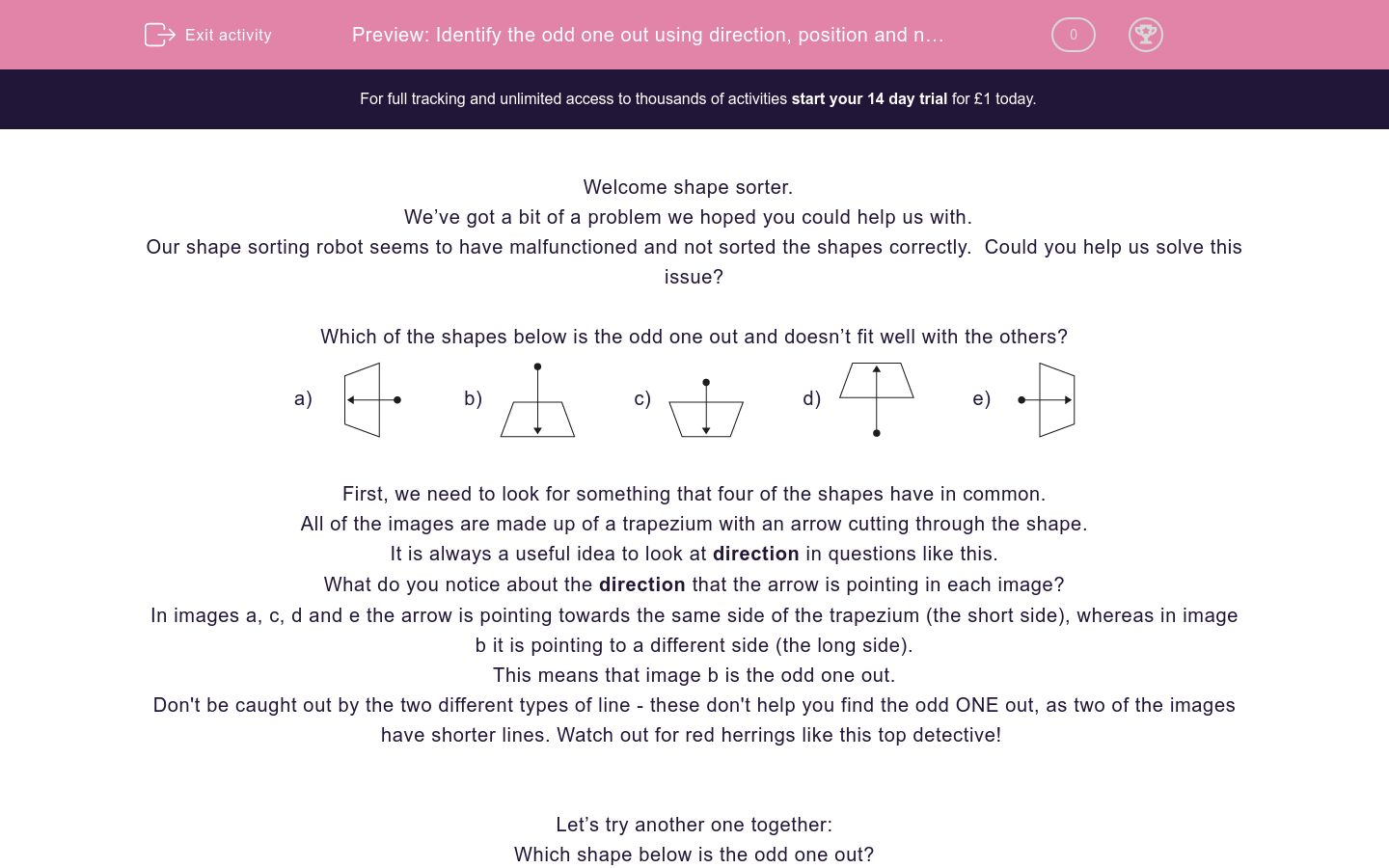Key stage:  KS 2

Curriculum topic:   Spatial and Non-Verbal Reasoning

Curriculum subtopic:   Odd One Out

Difficulty level:### QUESTION 1 of 10

Welcome shape sorter.

We’ve got a bit of a problem we hoped you could help us with.

Our shape sorting robot seems to have malfunctioned and not sorted the shapes correctly.  Could you help us solve this issue?

Which of the shapes below is the odd one out and doesn’t fit well with the others?

a)b)c)d)e)First, we need to look for something that four of the shapes have in common.

All of the images are made up of a trapezium with an arrow cutting through the shape.

It is always a useful idea to look at direction in questions like this.

What do you notice about the direction that the arrow is pointing in each image?

In images a, c, d and e the arrow is pointing towards the same side of the trapezium (the short side), whereas in image b it is pointing to a different side (the long side).

This means that image b is the odd one out.

Don't be caught out by the two different types of line - these don't help you find the odd ONE out, as two of the images have shorter lines. Watch out for red herrings like this top detective!

Let’s try another one together:

Which shape below is the odd one out?

a)b)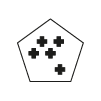c)d)e)First, we need to look for something that four of the shapes have in common.

In questions like this, counting is really important. What could we count?

We have two things to count here: the number of mini shapes and the number of sides of the main shape.

Image a is made up of a 3-sided shape with 3 crosses inside.

Image b is a 5-sided shape with 5 crosses inside.

Image c is a 4-sided shape with 4 crosses inside.

Image d is a 6-sided shape with 5 crosses inside.

Image e is a 4-sided shape with 4 crosses inside.

This means that image d is the odd one out as the number of sides of the main shape does not match the number of mini shapes inside, unlike the other options.

It’s now your turn to identify the odd image.

Remember to look at the direction and position of shapes within the image and always see if there is something you can count.

Good luck shape sorter!

Which of the images below is the odd one out?

a)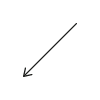b)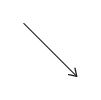c)d)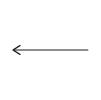e)Which of the images below is the odd one out?

a)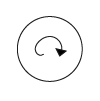b)c)d)e)Which of the images below is the odd one out?

a)b)c)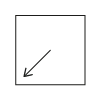d)e)Which of the images below is the odd one out?

a)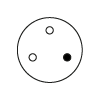b)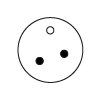c)d)e)Which of the images below is the odd one out?

a)b)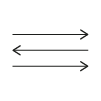c)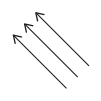d)e)Which of the images below is the odd one out?

a)b)c)d)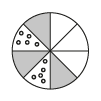e)Which of the images below is the odd one out?

a)b)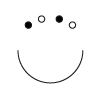c)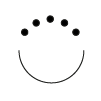d)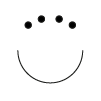e)Which of the images below is the odd one out?

a)b)c)d)e)Which of the images below is the odd one out?

a)b)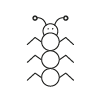c)d)e)Which of the images below is the odd one out?

a)b)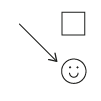c)d)e)• Question 1

Which of the images below is the odd one out?

d)EDDIE SAYS
This question is about direction as all the arrows look the same size. In options a, b, c and e the arrow is pointing diagonally either up or down, but in option d it is flat and pointing horizontally. This makes option d the one odd out.
• Question 2

Which of the images below is the odd one out?

c)EDDIE SAYS
It's another direction question top detective! In options a, b, d and e the arrows are pointing in a clockwise direction, but in option c the arrow is pointing in an anti-clockwise direction. This makes option c the odd one out. Remember that clockwise means in the same direction as the movement of the hands on the face of a clock.
• Question 3

Which of the images below is the odd one out?

e)EDDIE SAYS
Don't worry if you missed the POINT in this question - the arrows were the clue you were looking for here. In options a, b, c and d the arrows are pointing to a corner of the square. In option e the arrow is pointing to a side, rather than a corner. This makes option e the odd one out. Remember that the corner of a square is always a right angle or 90 degrees.
• Question 4

Which of the images below is the odd one out?

c)EDDIE SAYS
This one is a counting-focused question, as all the outer circles are the same so they don't help us find the right answer. We have a choice of whether to count the small white circles, the small black circles or the total number of circles. We need to look at the total number to reach the correct answer. In options a, b, d and e there are 3 small circles inside the larger outer circle, but in option c there are 4. This makes option c the odd one out.
• Question 5

Which of the images below is the odd one out?

b)EDDIE SAYS
Arrows, arrows everywhere! In four of these images. all the 3 arrows are pointing in the same direction but in one they are not. Can you spot the option where the arrows are pointing in different directions? It's option b as the top and bottom are pointing to the right, but the middle arrow is pointing to the left. This makes option b the odd one out. Now this would be a very confusing road sign!
• Question 6

Which of the images below is the odd one out?

d)EDDIE SAYS
It's counting time again! All of the circles have the same number of total segments (8) so we need to look at the combination of shaded, blank and dotty segments. All of the circles have 4 blank segments, so we need to look at the dotty and shaded segments. Options a, b, c and e have 2 shaded segments and 2 spotty segments. Greedy option d has 3 shaded segments and 2 spotty segments, making it the odd one out.
• Question 7

Which of the images below is the odd one out?

c)EDDIE SAYS
Let's take a look at these creepy monsters with so many eyes! We need to count the number of googly eyes they have. Monsters a, b, d and e have 4 googly eyes, but monster c has 5! This makes monster c the odd one out. You may notice that some of their eyes are black and some are white - this is interesting to observe but doesn't help us answer the question.
• Question 8

Which of the images below is the odd one out?

b)EDDIE SAYS
Try not to get mesmerised looking into these shapes! This is a counting-themed question. Options a, c, d and e are made up of three layers of the same shape, however, if you look carefully you can see that option b has 4 layers. This makes option b the odd one out. Can you name all the shapes here top shape sorter?
• Question 9

Which of the images below is the odd one out?

c)EDDIE SAYS
Try not to get itchy at the sight of these bugs! If we look carefully we can see that bugs a, b, d and e all have 3 sections to their body, but bug c has 4. This makes option c the odd one out.
• Question 10

Which of the images below is the odd one out?

e)EDDIE SAYS
Where is the arrow pointing in each of these options? In options a, b, c and d the arrow is pointing to the smiley face, but in option e it is pointing to the square. This makes option e the odd one out. Always remember top detective that arrows are often a great clue which POINT you in the direction of the correct answer.
---- OR ----